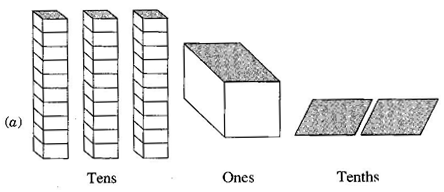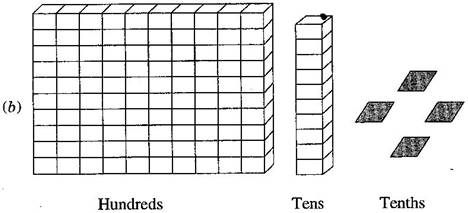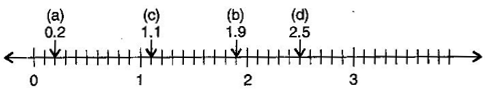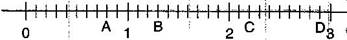1. /
2. CBSE
3. /
4. Class 06
5. /
6. Mathematics
7. /
8. NCERT Solutions for Class...

# NCERT Solutions for Class 6 Maths Exercise 8.1

NCERT solutions for Maths Decimals## NCERT Solutions for Class 6 Maths Decimals

###### Question 1.Write the following as numbers in the given table:Hundreds(100) Tens(10) Ones(1) Tenths{tex}\left( {\frac{1}{{10}}} \right){/tex}

 Hundreds(100) Tens(10) Ones(1) Tenths{tex}\left( {\frac{1}{{10}}} \right){/tex} 0 3 2 31.2 1 1 4 110.4
###### Question 2.Write the following decimals in the place value table:

(a) 19.4

(b) 0.3

(c) 10.6

(d) 205.9

(a)

 Hundreds Tens Once Tenths 0 1 9 4

(b)

 Hundreds Tens Once Tenths 0 0 0 3

(c)

 Hundreds Tens Once Tenths 0 1 0 6

(d)

 Hundreds Tens Once Tenths 0 0 5 9

NCERT Solutions for Class 6 Maths Exercise 8.1

###### Question 3.Write each of the following as decimals:

(a) seven-tenths

(b) Two tens and nine-tenths

(c) Fourteen point six

(d) One hundred and two-ones

(e) Six hundred point eight

(a) seven-tenths = 7 tenths = {tex}\frac{7}{{10}}{/tex} = 0.7

(b) 2 tens and 9-tenths = 2 x 10 + {tex}\frac{9}{{10}}{/tex} = 20 + 0.9 =20.9

(c) Fourteen point six = 14.6

(d) One hundred and 2-ones = 100 + 2 x 1 = 100 + 2 = 102

(e) Six hundred point eight = 600.8

###### Question 4.Write each of the following as decimals:

(a) {tex}\frac{5}{{10}}{/tex}

(b) {tex}3 + \frac{7}{{10}}{/tex}

(c) {tex}200 + 60 + 5 + \frac{1}{{10}}{/tex}

(d) {tex}70 + \frac{8}{{10}}{/tex}

(e) {tex}\frac{{88}}{{10}}{/tex}

(f) {tex}4\frac{2}{{10}}{/tex}

(g) {tex}\frac{3}{2}{/tex}

(h) {tex}\frac{2}{5}{/tex}

(i) {tex}\frac{{12}}{5}{/tex}

(j) {tex}3\frac{3}{5}{/tex}

(k) {tex}4\frac{1}{2}{/tex}

(a) {tex}\frac{5}{10}{/tex} = 0.5

(b) {tex}3+\frac{7}{10}{/tex} = 3 + 0.7 = 3.7

(c) {tex}200+60+5+\frac{1}{10}{/tex} = 200 + 60 + 5 + 0.1 = 265.1

(d) {tex}70+\frac{8}{10}{/tex} = 70 + 0.8 = 70.8

(e) {tex}\frac{88}{10}=\frac{80+8}{10}=\frac{80}{10}+\frac{8}{10}=8+\frac{8}{10}\partial {/tex}= 8 + 0.8 = 8.8

(f) {tex}4\frac{2}{10}=4+\frac{2}{10}{/tex} = 4 + 0.2 = 4.2

(g) {tex}\frac{3}{2}=\frac{3\times 5}{2\times 5}=\frac{15}{10}=\frac{10+5}{10}=\frac{10}{10}+\frac{5}{10}{/tex} = 1 + 0.5 = 1.5

(h) {tex}\frac{2}{5}=\frac{2\times 2}{5\times 2}=\frac{4}{10}{/tex} = 0.4

(i) {tex}\frac{12}{5}=\frac{12\times 2}{5\times 2}=\frac{24}{10}=\frac{20+4}{10}=\frac{20}{10}+\frac{4}{10}{/tex} = 2 + 0.4 = 2.4

(j) {tex}3\frac{3}{5}=3+\frac{3}{5}=3+\frac{3\times 2}{5\times 2}=3+\frac{6}{10}{/tex} = 3 + 0.6 = 3.6

(k) {tex}4\frac{1}{2}=4+\frac{1}{2}=4+\frac{1\times 5}{2\times 5}=4+\frac{5}{10}{/tex} = 4 + 0.5 = 4.5

NCERT Solutions for Class 6 Maths Exercise 8.1

###### Question 5.Write the following decimals as fraction. Reduce the fractions to lowest terms:

(a) 0.6

(b) 2.5

(c) 1.0

(d) 3.8

(e) 13.7

(f) 21.2

(g) 6.4

(a) 0.6 = {tex}\frac{6}{10}=\frac{3}{5}{/tex}

(b) 2.5 = {tex}\frac{25}{10}=\frac{5}{2}{/tex}

(c) 1.0 = {tex}\frac{10}{10}{/tex} = 1

(d) 3.8 = {tex}\frac{38}{10}=\frac{19}{5}{/tex}

(e) 13.7 = {tex}\frac{137}{10}{/tex}

(f) {tex}21.2=\frac{212}{10}{/tex} = {tex}\frac{106}{5}{/tex}

(g) 6.4 = {tex}\frac{64}{10}=\frac{32}{5}{/tex}

###### Question 6.Express the following as cm using decimals:

(a) 2 mm

(b) 30 mm

(c) 116 mm

(d) 4 cm 2 mm

(e) 162 mm

(f) 83 mm

(a) {tex}\because {/tex} 10 mm = 1 cm

{tex}\therefore {/tex} 1 mm = {tex}\frac{1}{10}{/tex} cm

{tex}\therefore {/tex} 2 mm = {tex}\frac{1}{10}{/tex} x 2 = 0.2 cm

(b) {tex}\because {/tex} 10 mm = 1 cm

{tex}\therefore {/tex} 1 mm = {tex}\frac{1}{10}{/tex} cm

{tex}\therefore {/tex} 30 mm = {tex}\frac{1}{10}{/tex} x 30 = 3.0 cm

(c) {tex}\because {/tex} 10 mm = 1 cm

{tex}\therefore {/tex} 1 mm = {tex}\frac{1}{10}{/tex} cm

{tex}\therefore {/tex} 116 mm = {tex}\frac{1}{10}{/tex} x 116 = 11.6 cm

(d) 4 cm + {tex}\frac{2}{10}{/tex} cm [{tex}\because {/tex} 10 mm = 1 cm]

4 + 0.2 = 4.2 cm

(e) {tex}\because {/tex} 10 mm = 1 cm

{tex}\therefore {/tex} 1 mm = {tex}\frac{1}{10}{/tex} cm

{tex}\therefore {/tex} 162 mm = {tex}\frac{1}{10}{/tex} x 162 = 16.2 cm

(f) {tex}\because {/tex} 10 mm = 1 cm

{tex}\therefore {/tex} 1 mm = {tex}\frac{1}{10}{/tex} cm

{tex}\therefore {/tex} 83 mm = {tex}\frac{1}{10}{/tex} x 83 = 8.3 cm

NCERT Solutions for Class 6 Maths Exercise 8.1

###### Question 7.Between which two whole numbers on the number line are the given lie? Which of these whole numbers is nearer the number?

(a) 0.8

(b) 5.1

(c) 2.6

(d) 6.4

(e) 9.1

(f) 4.9

(a) From 0 to 1, 0.8 is nearest to 1.

(b) From 5 to 6, 5.1 is nearest to 5.

(c) From 2 to 3, 2.6 is nearest to 3.

(d) From 6 to 7, 6.4 is nearest to 6.

(e) From 9 to 10, 9.1 is nearest to 9.

(f) From 4 to 5, 4.9 is nearest to 5.

###### Question 8.Show the following numbers on the number line:

(a) 0.2

(b) 1.9

(c) 1.1

(d) 2.5Question 9.Write the decimal number represented by the points A, B, C, D:A = 0 + {tex}\frac{8}{{10}}{/tex} = 0.8

B = 1 + {tex}\frac{3}{{10}}{/tex} = 1.3

C = 2 + {tex}\frac{2}{{10}}{/tex} = 2.2

D = 2 + {tex}\frac{9}{{10}}{/tex} = 2.9

NCERT Solutions for Class 6 Maths Exercise 8.1

###### Question 10.

(a) The length of Ramesh’s notebook is 9 cm and 5 mm. What will be its length in cm?

(b) The length of a young gram plant is 65 mm. Express its length in cm.

(a) 9 cm 5 mm = 9 cm + 5 mm = 9 + {tex}\frac{5}{{10}}{/tex} = 9.5 cm

(b) 65 mm = {tex}\frac{{65}}{{10}}{/tex} cm = 6.5 cm.

## NCERT Solutions for Class 6 Maths Exercise 8.1

NCERT Solutions Class 6 Maths PDF (Download) Free from myCBSEguide app and myCBSEguide website. Ncert solution class 6 Maths includes text book solutions from Class 6 Maths Book . NCERT Solutions for CBSE Class 6 Maths have total 14 chapters. 6 Maths NCERT Solutions in PDF for free Download on our website. Ncert Maths class 6 solutions PDF and Maths ncert class 6 PDF solutions with latest modifications and as per the latest CBSE syllabus are only available in myCBSEguide.

## CBSE app for Students

To download NCERT Solutions for Class 6 Maths, Social Science Computer Science, Home Science, Hindi English, Maths Science do check myCBSEguide app or website. myCBSEguide provides sample papers with solution, test papers for chapter-wise practice, NCERT solutions, NCERT Exemplar solutions, quick revision notes for ready reference, CBSE guess papers and CBSE important question papers. Sample Paper all are made available through the best app for CBSE students and myCBSEguide website.### Test Generator

Create question paper PDF and online tests with your own name & logo in minutes.### myCBSEguide

Question Bank, Mock Tests, Exam Papers, NCERT Solutions, Sample Papers, Notes

### 2 thoughts on “NCERT Solutions for Class 6 Maths Exercise 8.1”

1. i like this website very much.it is so helpful for me

2. Very nice